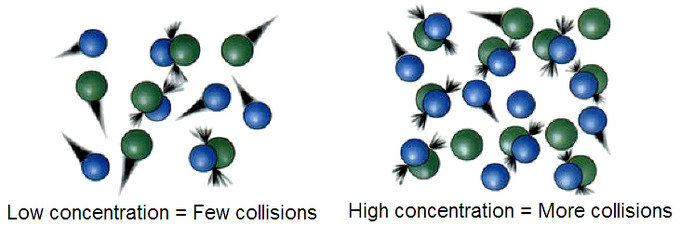# Collision Theory Study Guide

INTRODUCTION

Collision theory in chemistry allows scientists to predict a rate constant of a chemical reaction which can be used to determine reaction rates. This theory was developed by Max Trautz and William Lewis to derive meaningful insights into these chemical reactions.

### WHAT IS COLLISION THEORY

Collision theory states that the rate of a chemical reaction is directly proportional to the number of collisions occurring between the reactant molecules. In simple terms, the collision theory in chemistry explains what initiates chemical reactions. Theoretically, the more that the reactant molecules collide with one another, the faster the rate of reaction becomes. In reality, however, only a small fraction of the collisions are what we term effective collisions, which are the only type of collision that results in the creation of a product.

To initiate effective collisions, reactant molecules are required to have activation energy, which is the minimum amount of energy required to undergo a reaction. The count of molecules possessing sufficient energy is all dependent on the reactant’s temperature. If these reactants do not possess their required activation energy, they bounce back from each other without even reacting.

### Temperature Dependency of Collision Theory

According to collision theory, the reaction happens between the active molecules. The percentage of activated molecules and the percentage of activated collisions both climb as temperature rises. As a result, the rate of reaction rises.

The activation energy is returned in the form of vibrational energy when the reaction is finishing and the products are forming. This energy is immediately discharged as heat. Therefore, it is crucial that the molecules collide at energies larger than or equivalent to the reaction’s activation energy.

Applying the Arrhenius equation to two different temperatures yields the same result (T1andT2)Log k2/k1=Ea/2.303R(T2–T1/T1T2)

Where k1 = specific rate at T1

k2 = specific rate at T2Ea = activation energyR = gas constant

### Molecular Collision

• The simple rule is that there will be more collisions the more molecules there are. As a result, depending on how many particles collide, different proportions of collisions occur.
• Before molecules may react chemically, they must first collide.
• Kinetic energy needs be present in collisions to initiate a reaction. A strong enough contact strength is required to break the binding.
• Temperature has an impact on collisions; the higher the temperature, the more collisions. The severity of crashes increases with temperature.
• In contrast to molecules with sufficient energy, neutral molecules cannot break any bonds or take part in the collision process since they have a lower energy level.
• The reaction process involves the bond being bent, stretched, and twisted. As a result, the procedure needs energetic molecules.

### POSTULATES OF COLLISION THEORY

Collision theory is based on three postulates:The rate of reaction is proportional to the rate of reactant collision molecules. The reacting element should collide to allow contact between the atoms, which are meant to be bonded together. There must be sufficient energy to allow mutual penetration of the reactants so that the electrons can again arrange and form new bonds.

## CONCLUSION

• Collision theory occurs at certain chemical reactions and rates.
• The fundamental principle is that in order to react, molecules must undergo effective collisions.
• In order to undergo an effective collision, the molecules’ collision energy must be greater than or equal to activation energy during the reaction.
• Reactants with low concentration result in few collisions, and those with high concentrations produce more collisions.

### FAQs

1. What is collision theory?

Collision theory defines that the chemical reaction rate is proportional to the number of collisions occurring between the reactant molecules. This occurs at certain chemical reactions and rates.

2. What are the 4 points of collision theory?

• Reactants must collide in the proper orientation.
• Collision energy must be greater than or equal to activation energy during the reaction.
• The reactant particles must collide with one another.
• The collision frequency should be larger than the number of factors for the reaction.

3. What are the three postulates of collision theory?

• The rate of reaction is proportional to the rate of reactant collision molecules.
• The reacting molecules must collide to allow contact between the atoms, which are meant to bond together.
• There should be sufficient energy to allow joint penetration of the reactants so that the electrons can rearrange and form new bonds.

4. What equation is used for collision theory?

K ∝ e-Ea/RT

K = A e-Ea/RT

where, A is the frequency factor, Ea is the activation energy, R is gas constant and T is the absolute temperature

This is the Arrhenius equation, and the collision theory equation is accommodated in this only.We hope you enjoyed studying this lesson and learned something cool about Collision Theory! Join our Discord community to get any questions you may have answered and to engage with other students just like you! Don’t forget to download our app to experience our fun VR classrooms – we promise it makes studying much more fun! 😎

]]>